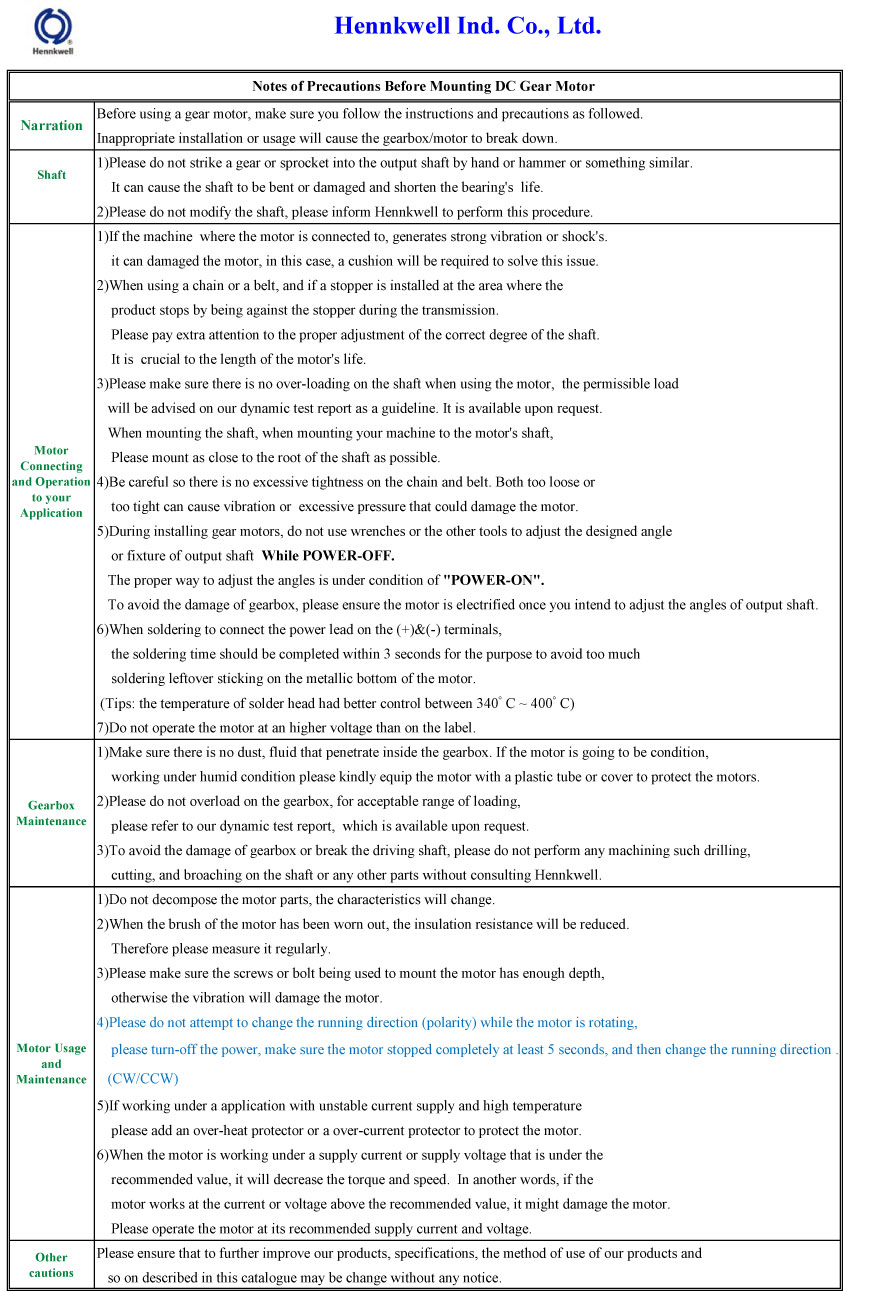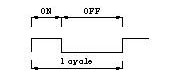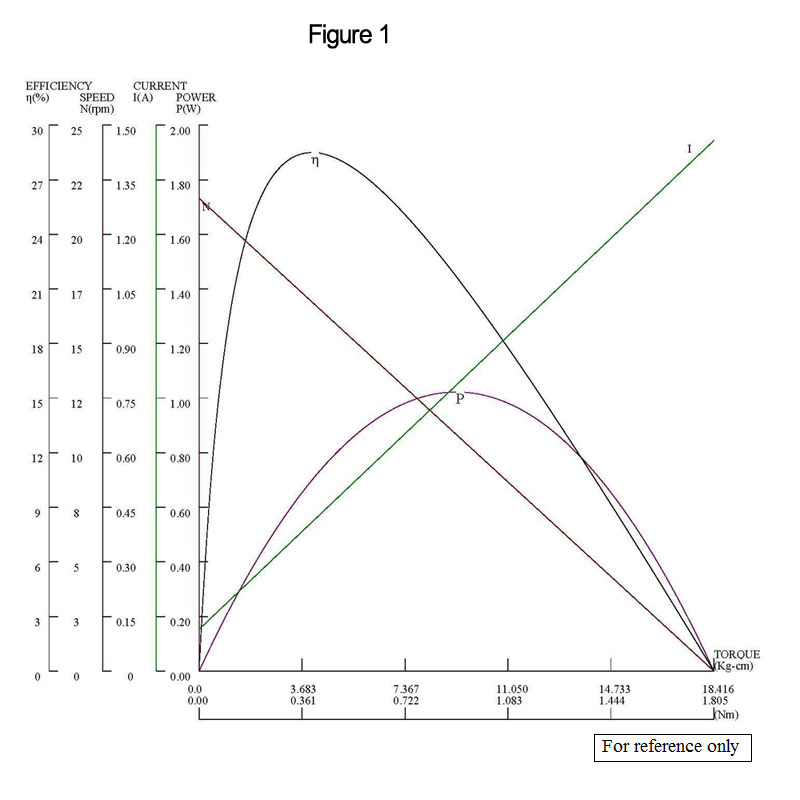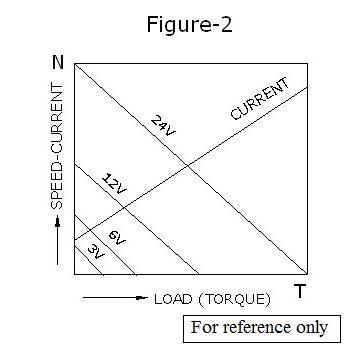## Hennkwell Introduction

Hennkwell Ind. Co., Ltd. is Taiwan supplier and manufacturer in Mechanical Components Industry. Hennkwell has been offering our customers high quality Gear Motor, Geared Motor, 12V DC Gear Motor, Dc Gear Motor, 24V DC Gear Motor, DC Geared Motor, 12V DC Motor with Gear Reduction, Dc Motor with Gearbox 24V, Gear Box DC Motor, Planetary Gearbox since 1995. With both advanced technology and 20 years experience, Hennkwell always make sure to meet each customer's demand.

### FAQ

Result 1 - 3 of 3
##### Notes of Precautions Before Mounting DC Gear Motor##### Unit Conversions

Torque:
1 kgf.cm = 13.8874 oz.in = 0.867962 lb.in = 9.8 mNm = 0.07233 lb.ft = 1000 gf.cm = 9.8 Ncm
1 oz.in = 0.0720 kgf.cm = 0.0625 lb.in = 7.06155 mNm
1 lb.in = 1.15212 kgf.cm = 16 oz.in = 112.985 mNm
1 mNm = 0.010197 kgf.cm = 0.1416 oz.in = 0.8851 lb.in

Length:
1 inch = 2.54 cm = 25.4 mm = 0.00833 ft
1 mm = 0.0394 inch = 0.0033 ft = 1000 μ
1 ft = 12 inches = 304.8 mm = 30.48 cm

Weight:
1 kg = 1000 g = 2.205 lb = 35.28 oz
1 oz = 0.0283 kg = 0.0625 lb = 28.35 g
1 lb = 0.4536 kg = 16 oz = 453.6 g

Temperature:
T(℃) Celsius = 5/9 T (℉)-32
T(℉) Fahrenheit = 9/5 T (℃)+32

##### Terminology for the Gear Motor
1. Rated Voltage: The specific voltage required by the gear motor under normal working conditions for which its optimal performance is displayed. Typically, the power supply voltage should not vary by more than 10% of the motor's rated voltage so as not to negatively affect the speed, current, torque, and temperature. An operation requiring over-voltage will result in a higher current running through but the gear motor may experience a shortened life span. An under-voltage operation will not be running at peak levels resulting in experiences of lower current, efficiency, and torque. Therefore, maintenance of the proper voltage is an important factor in obtaining good performance.
2. No-Load Speed: The revolutions per minute with no load applied to the drive shaft. This is linearly proportional to the applied voltage.
3. Rated Speed: The most favorable speed (rpm) of the gear motor shall be a rated voltage and rated output torque.
4. No-Load Current: Current drawn at rated voltage under no-load conditions. The current is caused by internal mechanical friction losses occurring between the brush and commutator segments as well as the bush/bearing and shaft friction.
5. Rated Torque: The rated torque is a loaded turning force under rated voltage. The applied gear motor may be operated only at the allowable level. Operation at a load higher than rated torque for the gear motor is never recommended.
6. Starting Torque: The torque delivered by a motor at the instant and maximum current. Starting torque is much higher than rated running or full load torque.
7. Duty Cycle: The relationship between the operating and rest time, or repeatable operation at different load. Duty cycle in percentage values is equal to the ON-time divided by the sum of the On-time plus the Off-time x 100%. The DC brush gear motor can operate under intermittent or continuous duty within the temperature limits. But, most micro DC brush gear motors are often used within a short time of intermittent duty.
8.9. Duty Cycle(%)= On-time ÷ (On-time ＋ off-time) × 100%
Maximum Allowable Torque
: The running torque can be increased in accordance with the bigger gearbox reduction ratio. However, the practical limitation of loading torque shall be affected by the gear material, rising temperature, and some other conditions. Please refer to the gearbox specifications on page.
10. Gear Train Transmission Efficiency: Expressed in percentage values, the transmission efficiency determines the friction between the bush and gears as well as the resistance of lubricant, etc. The efficiency is about 81% when the gear ratio is in the first gear section and 73% in the second section; that is, as the gear (reduction) ratio becomes greater, the section number increases and the transmission efficiency decreases by 66%, 59%, 53%, and 48%.
11. Radial Load: A force pushing or pulling the side of side of the output shaft. If the force exceeds the allowable radial load for the motor or gear motor, it will cause the output shaft to break and cause premature wear of the output shaft bearing/bush as well as the gears.
12. Axial Load: A force on the output shaft into or out of the motor or gear motor. If the force exceeds the allowable axial load for the motor or gear motor, it will cause premature wear of the output shaft bearing and gearing.
##### DC Gear Motor Characteristics

The relationship between torque (T), speed (N), current (I), efficiency (E), and output power (P), as below figures shown, represents the characteristics of a Hennkwell micro DC gear motor. Figure-1 shows how the loaded torque on the gear motor is in proportion and is directly related to the output speed and current. Figure-2 shows how the no-load speed and starting torque also change proportionally to a different supply voltage. The output speed at a given voltage is parallel to those at other voltage.

As the load on the gear motor increases, speed will decrease accordingly. Additionally, the current (I) is an inverse relationship to torque. The peak of output power (P) and efficiency (E) exist at different torque points, as indicated in figure-1. Output power presents a curve by torque while efficiency (E) decreases straight down beyond the peak normally. Maximum output (Pmax) is at half the starting torque (Ts) point and maximum efficiency exists at a much lower point of torque. A gear motor's basic rating point is lower than its maximum efficiency point. Load torque can be determined by measuring the current drawn when the gear motor is installed in a machine where actual load value is known.

Moreover, the actual operating load torque must be selected lower by a few times than the stall torque. The purpose is to prolong the motor’s life and to bring out the most optimal performance. Besides the full load torque has to operate within the maximum allowable torque limit, although the gear motor could produce an over torque.

The maximum efficiency is generally much lower than the maximum stalled torque, because although the motor can operate at a higher torque than the maximum efficiency torque, it can also shorten the motor’s life due to high current generated. Thus, selecting a motor with a torque that is several times higher than the actual operating torque is strongly recommended.Result 1 - 3 of 3